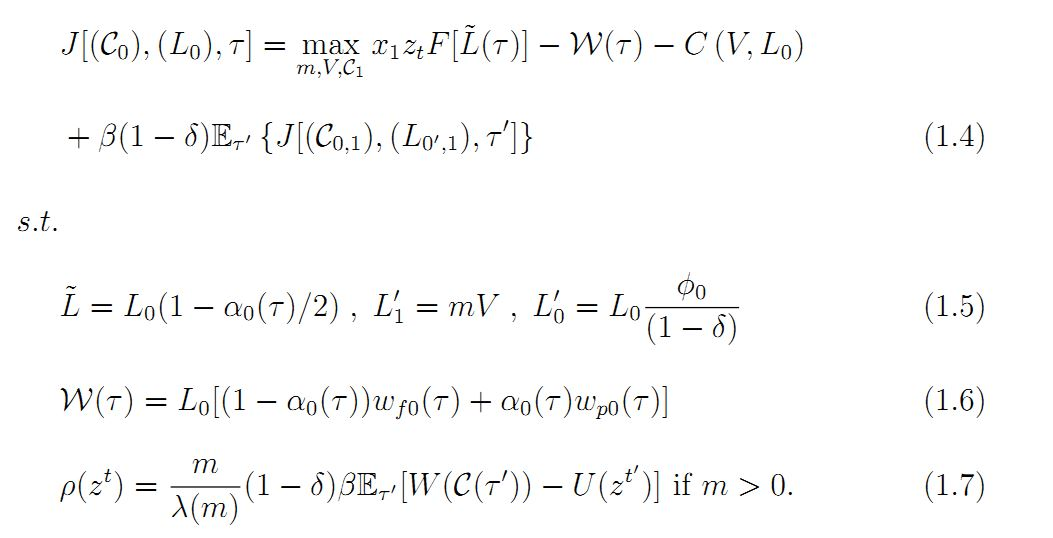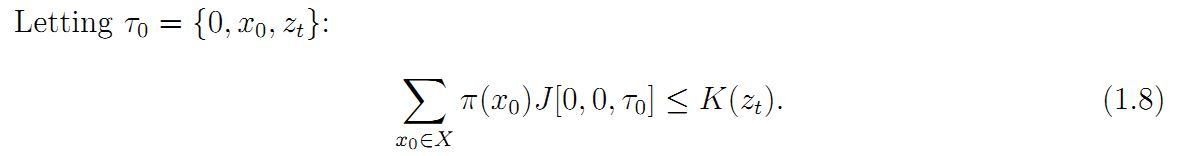# The Firm’s Problem at Age 1

The firm’s problem involves the recruitment of new workers through the post­ing of contracts for new vacancies, and the commitment to past contracts offered to its current labor force. The firm of age j in history (xj, zt) takes as given its current stock of workers hired up to the current period, ( Li ) ij=01 , and the contracts signed by previous labor cohorts, ( Ci ) ij=01 . The firm’s separation rate and retention probability must satisfy s(t) = (1-ộ(t))/(1-Ổ) in order to avoid violating its prior commitments to past labor cohorts, and to be consistent with exogenous probabilities of separation and firm death. The firm then decides to post a contract Cj in V vacancies. The contract Cj achieves the firm’s desired matching probability m, taking the worker’s value of search p(zt) as given. To simplify, I describe the problem of a firm of age 1 with a single cohort of existing workers. The complete firm’s problem and definition of competitive equilibrium appears in Appendix A.1.

Consider a firm of age 1 in history (x1, zt). Letting t = {1, (x1, zt)}, the problem of the age 1 firm is:The firm enters the period with an existing labor force Lo hired under contracts Co.

The firm produces using L in (1.5) and pays a wage bill W in (1.6), with a and {wf, wp} being consistent with contract Co. The firm chooses contracts C1 to post in V vacancies at a cost C(V, L). It chooses a matching probability m through the value of the contracts posted, knowing that achieving m requires a queue length of A(m) per vacancy. The minimum utility a contract C must promise to attract a queue length of A(m) per vacancy comes from the worker’s participation constraint, (1.7). At the end of the period, the firm’s current cohort separates at rate s = leaving lo = Lo(1 – s) remaining in that cohort. The firm then gains a new cohort L1 = mV from recruitment (1.5). This problem generalizes to the firm of age j, where each cohort and contract is indexed by the age of the firm at recruitment. The total labor force and wage bill are similar, except that they consist of the sum of all cohorts and their respective contracts. Lastly, the firm’s policies must be consistent with its commitment to past cohorts.

Free entry of firms implies that the expected value of an entrant firm (before realizing x and with labor force L = 0) is less than or equal to the entry cost K (zt).If entry is positive, then this condition holds with equality.